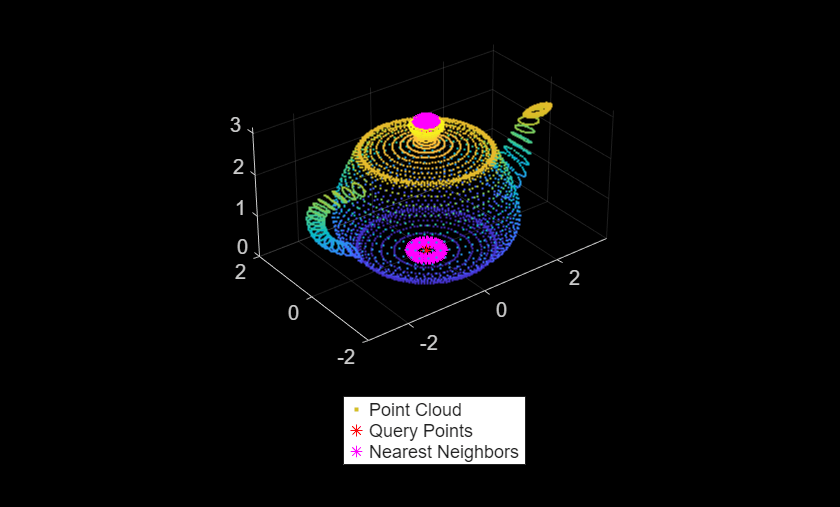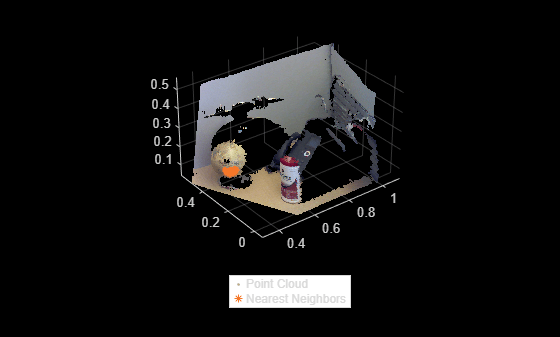# findNearestNeighbors

Find nearest neighbors of a point in point cloud

## Syntax

``[indices,dists] = findNearestNeighbors(ptCloud,point,K)``
``[indices,dists] = findNearestNeighbors(ptCloud,point,K,camMatrix)``
``[indices,dists] = findNearestNeighbors(___,Name,Value)``

## Description

example

````[indices,dists] = findNearestNeighbors(ptCloud,point,K)` returns the `indices` for the K-nearest neighbors of a query point in the input point cloud. `ptCloud` can be an unorganized or organized point cloud. The K-nearest neighbors of the query point are computed by using the Kd-tree based search algorithm. ```

example

````[indices,dists] = findNearestNeighbors(ptCloud,point,K,camMatrix)` returns the K-nearest neighbors of a query point in the input point cloud. The input point cloud is an organized point cloud generated by a depth camera. The K-nearest neighbors of the query point are determined using fast approximate K-nearest neighbor search algorithm. The function uses the camera projection matrix `camMatrix` to know the relationship between adjacent points and hence, speeds up the nearest neighbor search. However, the results have lower accuracy as compared to the Kd-tree based approach. NoteThis syntax only supports organized point cloud data produced by RGB-D sensors.You can use `estimateCameraMatrix` to estimate camera projection matrix for the given point cloud data. ```
````[indices,dists] = findNearestNeighbors(___,Name,Value)` specifies options using one or more name-value arguments in addition to the input arguments in the preceding syntaxes.```

## Examples

collapse all

Load a set of 3-D coordinate points into the workspace.

`load('xyzPoints.mat');`

Create a point cloud object.

`ptCloud = pointCloud(xyzPoints);`

Specify a query point and the number of nearest neighbors to be identified.

```point = [0,0,0]; K = 220;```

Get the indices and the distances of K nearest neighboring points.

`[indices,dists] = findNearestNeighbors(ptCloud,point,K);`

Display the point cloud. Plot the query point and their nearest neighbors.

```figure pcshow(ptCloud) hold on plot3(point(1),point(2),point(3),'*r') plot3(ptCloud.Location(indices,1),ptCloud.Location(indices,2),ptCloud.Location(indices,3),'*') legend('Point Cloud','Query Point','Nearest Neighbors','Location','southoutside','Color',[1 1 1]) hold off```Find the K-nearest neighbors of a query point in the organized point cloud data by using the camera projection matrix. Compute the camera projection matrix from sampled point cloud data points and their corresponding image point coordinates.

Load an organized point cloud data into the workspace. The point cloud is generated by using the Kinect depth sensor.

```ld = load('object3d.mat'); ptCloud = ld.ptCloud;```

Specify the step size for sampling the point cloud data.

`stepSize = 100;`

Sample the input point cloud and store the sampled 3-D point coordinates as a point cloud object.

```indices = 1:stepSize:ptCloud.Count; tempPtCloud = select(ptCloud,indices);```

Remove invalid points from the sampled point cloud.

`[tempPtCloud,validIndices] = removeInvalidPoints(tempPtCloud);`

Define the 3-D world point coordinates of input point cloud.

`worldPoints = tempPtCloud.Location;`

Find the 2-D image coordinates corresponding to the 3-D point coordinates of input point cloud.

```[Y,X] = ind2sub([size(ptCloud.Location,1),size(ptCloud.Location,2)],indices); imagePoints = [X(validIndices)' Y(validIndices)'];```

Estimate camera projection matrix from the image and the world point coordinates.

`camMatrix = estimateCameraMatrix(imagePoints,worldPoints);`

Specify a query point and the number of nearest neighbors to be identified.

```point = [0.4 0.3 0.2]; K = 20;```

Find the indices and distances of K nearest neighboring points by using the camera projection matrix. Use the point cloud method `select` to get the point cloud data of nearest neighbors.

```[indices,dists] = findNearestNeighbors(ptCloud,point,K,camMatrix); ptCloudB = select(ptCloud,indices);```

Display the point cloud and the nearest neighbors of the query point.

```figure pcshow(ptCloud) hold on pcshow(ptCloudB.Location,'ob') legend('Point Cloud','Nearest Neighbors','Location','southoutside','Color',[1 1 1]) hold off```## Input Arguments

collapse all

Point cloud, specified as a `pointCloud` object.

Note

The function supports organized point cloud data generated only from RGB-D sensors.

Query point, specified as a three-element vector of form `[x,y,z]`.

Number of nearest neighbors, specified as a positive integer.

Camera projection matrix, specified as a 4-by-3 matrix that maps 3-D world points to 2-D image points. You can compute the `camMatrix` by using the `estimateCameraMatrix` function.

### Name-Value Arguments

Specify optional comma-separated pairs of `Name,Value` arguments. `Name` is the argument name and `Value` is the corresponding value. `Name` must appear inside quotes. You can specify several name and value pair arguments in any order as `Name1,Value1,...,NameN,ValueN`.

Example: `findNearestNeighbors(ptCloud,point,k,'Sort',true)`

Sort indices, specified as a comma-separated pair of `'Sort'` and a logical scalar. When you set `Sort` to `true`, the returned indices are sorted in the ascending order based on the distance from a query point. To turn off sorting, set `Sort` to `false`.

Number of leaf nodes to check, specified as a comma-separated pair consisting of `'MaxLeafChecks'` and an integer. When you set this value to `Inf`, the entire tree is searched. When the entire tree is searched, it produces exact search results. Increasing the number of leaf nodes to check increases accuracy, but reduces efficiency.

Note

The name-value argument `'MaxLeafChecks'` is valid only with Kd-tree based search method.

## Output Arguments

collapse all

Indices of stored points, returned as a column vector. The vector contains K linear indices of the nearest neighbors stored in the point cloud.

Distances to query point, returned as a column vector. The vector contains the Euclidean distances between the query point and its nearest neighbors.

 Muja, M. and David G. Lowe. "Fast Approximate Nearest Neighbors with Automatic Algorithm Configuration". In VISAPP International Conference on Computer Vision Theory and Applications. 2009. pp. 331–340.